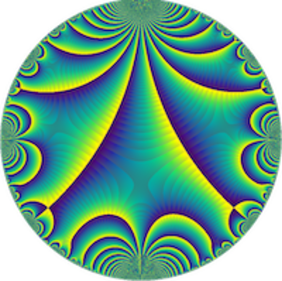# p-adic L-functions for GL(3) (Chris Williams, Université de Warwick)

Séminaire « Arithmétique »
M2 Kampé de Fériet

Let \pi be a p-ordinary cohomological cuspidal automorphic representation of GL(n,A_Q). A conjecture of Coates--Perrin-Riou predicts that the (twisted) critical values of its L-function L(\pi x\chi,s), for Dirichlet characters \chi of p-power conductor, satisfy systematic congruence properties modulo powers of p, captured in the existence of a p-adic L-function. For n = 1,2 this conjecture has been known for decades, but for n > 2 it is known only in special cases, e.g. symmetric squares of modular forms; and in all previously known cases, \pi is a functorial transfer via a proper subgroup of GL(n). In this talk, I will explain what a p-adic L-function is, state the conjecture more precisely, and then describe recent joint work with David Loeffler, in which we prove this conjecture for n=3 (without any transfer or self-duality assumptions).